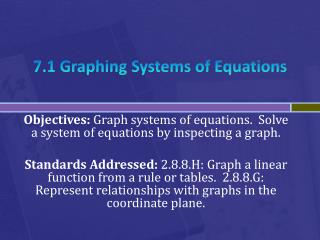DownloadDownload Presentation7.1 Graphing Systems of Equations

# 7.1 Graphing Systems of Equations

Download Presentation## 7.1 Graphing Systems of Equations

- - - - - - - - - - - - - - - - - - - - - - - - - - - E N D - - - - - - - - - - - - - - - - - - - - - - - - - - -
##### Presentation Transcript

1. 7.1 Graphing Systems of Equations Objectives: Graph systems of equations. Solve a system of equations by inspecting a graph. Standards Addressed: 2.8.8.H: Graph a linear function from a rule or tables. 2.8.8.G: Represent relationships with graphs in the coordinate plane.

2. Two equations in two variables are called a system of equation. • A solution to a system of equations is an ordered pair of numbers that is a solution to each equation in the system. • The solution to a system of linear equations can be found by graphing.